Worksheets and No Prep Teaching Resources
Math Worksheets

# Functions Math Worksheets

Any mathematician knows that he or she can't function without functions, and these unique worksheets will help students understand and master functions from very basic through higher level understanding of domain, range, inverse and compound functions, absolute value, transformation, and more. Detailed graphics and final review sheets make these worksheets especially effective so students understand, retain, and master this vital part of math.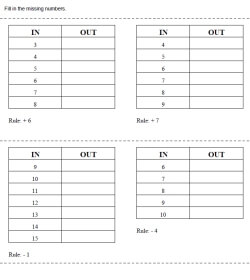Patterns and Functions: IN and OUT
Easier Functions: The Rule is Given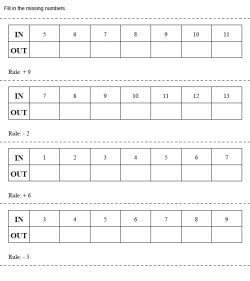Easy: Rule is given, IN are numbers that increase by 1, Fill in OUT, addition or subtraction, horizontal display Easy: Rule is given, IN are numbers that increase by 1, Some IN missing and some OUT, addition or subtraction, horizontal displayEasy: Rule is given, IN are numbers that increase by 1, Fill in OUT, addition or subtraction, vertical display Easy: Rule is given, IN are numbers that increase by 1, Some IN missing and some OUT, addition or subtraction, vertical display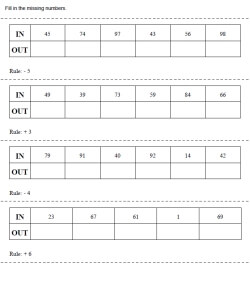Easy: Rule is given, IN values are random, Fill in OUT, addition or subtraction, horizontal display Easy: Rule is given, IN values are random, Some IN missing and some OUT, addition or subtraction, horizontal display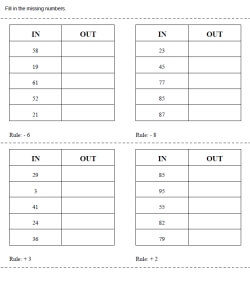Easy: Rule is given, IN values are random, Fill in OUT, addition or subtraction, vertical display Easy: Rule is given, IN values are random, Some IN missing and some OUT, addition or subtraction, vertical display

Rules Listed on Top of Page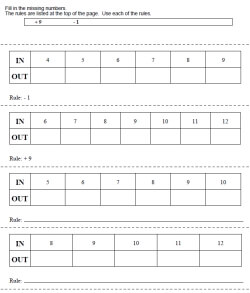Easy: Rule is given, IN are numbers that increase by 1, Fill in OUT, addition or subtraction, horizontal display Easy: Rule is given, IN are numbers that increase by 1, Some IN missing and some OUT, addition or subtraction, horizontal display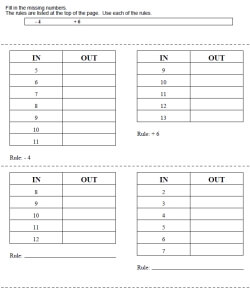Easy: Rule is given, IN are numbers that increase by 1, Fill in OUT, addition or subtraction, vertical display Easy: Rule is given, IN are numbers that increase by 1, Some IN missing and some OUT, addition or subtraction, vertical display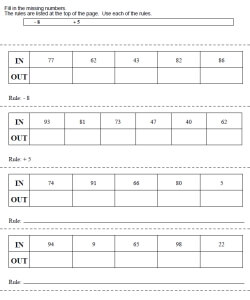Easy: Rule is given, IN values are random, Fill in OUT, addition or subtraction, horizontal display Easy: Rule is given, IN values are random, Some IN missing and some OUT, addition or subtraction, horizontal display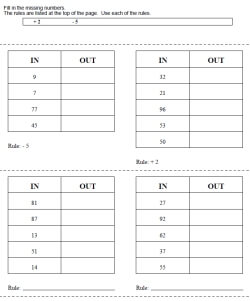Easy: Rule is given, IN values are random, Fill in OUT, addition or subtraction, vertical display Easy: Rule is given, IN values are random, Some IN missing and some OUT, addition or subtraction, vertical display

Patterns and Functions: IN and OUT with Shapes
Easier Functions: The Rule is Given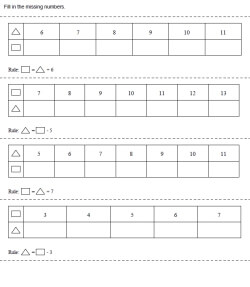Easy: Rule is given, IN are numbers that increase by 1, Fill in OUT, addition or subtraction, horizontal display Easy: Rule is given, IN are numbers that increase by 1, Some IN missing and some OUT, addition or subtraction, horizontal display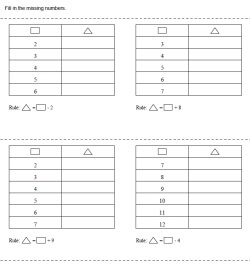Easy: Rule is given, IN are numbers that increase by 1, Fill in OUT, addition or subtraction, vertical display Easy: Rule is given, IN are numbers that increase by 1, Some IN missing and some OUT, addition or subtraction, vertical display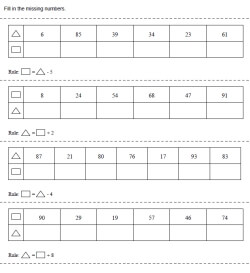Easy: Rule is given, IN values are random, Fill in OUT, addition or subtraction, horizontal display Easy: Rule is given, IN values are random, Some IN missing and some OUT, addition or subtraction, horizontal display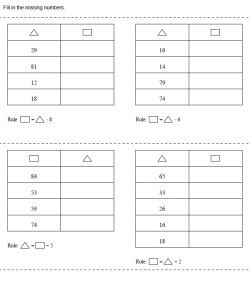Easy: Rule is given, IN values are random, Fill in OUT, addition or subtraction, vertical display Easy: Rule is given, IN values are random, Some IN missing and some OUT, addition or subtraction, vertical display

Algebra Functions Worksheets
Is the relation a function? (set of points)
Calculate the indicated value of f(x)
State the domain and range
State the domain and range (more difficult)
State whether the equation is a function
Given f(x) and g(x), calculate
Inverse (set of points)
State the inverse of each function
Verify that f and g are inverses of each other
Write a compound function for the absolute value function
Sketch the graph of the compound function
Identify the transformation from f(x) to g(x) - f(x) is always x2
Identify the transformation from f(x) to g(x)
Show how f(x) and g(x) are related
Final Review

Have a suggestion or would like to leave feedback?